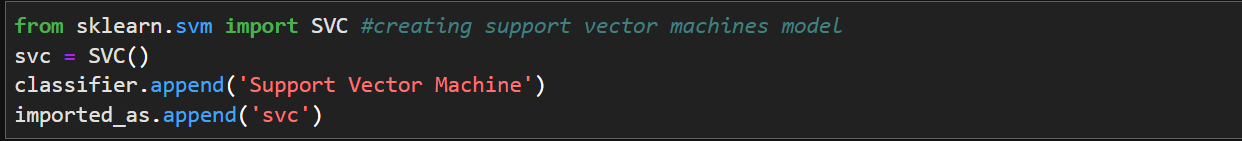## The Roadmap to Model Development Part 3

In last week’s blog post, we finished up the third step (Data Preprocessing for Prediction) and began creating our first models in the final step (Model Development). Today, we will create four more models, and test each of them to compare their accuracy and runtimes.

## Creating the models

Last blog post, we created only the Logistic Regression model and the K-Nearest Neighbors model. We will add the following four models today: Support Vector Machine, Decision Trees, Naive Bayes, and Random Forest. We will use the Sci-kit learn library for all of these.

• Support Vector MachineIn this step, we create the Support Vector Machine model and name it svc. We then append its full name to our ‘classifier’ array, and the instance to our ‘imported_as’ array. The Support Vector Machine model works by separating the data points into ‘hyperplanes’ that have optimal decision boundaries.

• Decision Tree

Here is the code implemented to create the Decision Tree model. It also appends the name and instance to the ‘classifier’ and ‘imported_as’ arrays, respectively. The Decision Tree model works by creating a ‘tree’ with multiple branches, with a binary question, leading to more branches.

• Naive Bayes

In this code block, we create the Naive Bayes model and append its name/instance to the ‘classifier’ and ‘imported_as’ arrays. Naive Bayes is a model that attempts to perform classification by assuming all features are independent of each other.

• Random Forest Classifier

This is the code to create the Random Forest Classifier Model. We then append it to the ‘classifier’ array and the ‘imported_as’ array. The Random Forest Classifier works by creating multiple decision trees and weighing all their results before a decision.

## Testing and measuring accuracy

We can now create our main class, that we will use to create an object with all the models so we can easily compare their accuracies.

We create a class called ‘Modeling’ in which we have a few functions, ‘fit’ and ‘results’ these two can be called on the ‘Modeling’ class object to fit the models, and to get the results, respectively. Now, we will make an array that holds all the models we want to test. We could also use the ‘imported_as’ array, but for simplicity we will make a new array and name it ‘models_to_test’.

Finally, we can create an object of the ‘Modeling’ class and use our ‘fit’ and ‘results’ functions on it. We will use our previously made arrays of x and y training data, x and y testing data, and our ‘models_to_test’ array as the arguments.

If it runs properly, you should be returned with ‘name of model’ has been fit for each of the models, and a pandas dataframe that holds the accuracy and runtime of each model.

The Random Forest Classifier seems to be the most accurate. If you want to test this multiple times to see if the RFC model is ever beat, you can use a simple for loop to get the top model as many times as you’d like.

You can run it as many times as you like, and you’ll see that the Random Forest Classifier is always the most accurate. However, this comes with a price of a significantly higher runtime than the other models (0.4 seconds vs 0.01 and lower). This is why we trained multiple models, so we could compare which one would deliver enough accuracy while still being appropriate for the hardware it will run on. From these models, you can choose which one is most appropriate for your use case.

## What’s next?

We now have a few accurate models to choose from for the purpose of predictive maintenance. However, the data used to train and test them is not our own. This project is meant to be easily adaptable to different sets of data, so next we will collect our own data for predictive maintenance and attempt to employ the model(s) on that dataset.